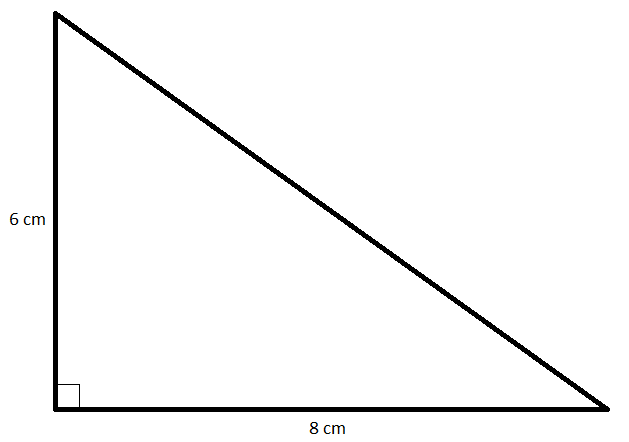# The two sides of a right-angled triangle are 6 cm and 8 cm. Find the length of the hypotenuse.

Given: The two sides of a right-angled triangle are 6 cm and 8 cm.

To find: Here we have to find the length of the hypotenuse.

Solution:We know that in a right-angled triangle:

(Hypotenuse)= (Base)$+$ (Height)2

Now,

Base = 8 cm and Height = 6 cm

(Hypotenuse)= (Base)$+$ (Height)2

(Hypotenuse)= 82 $+$ 62

(Hypotenuse)= 64 $+$ 36

(Hypotenuse)= 100

Hypotenuse = 10 cm

So, hypotenuse is equal to 10 cm.

Updated on: 10-Oct-2022

1K+ Views